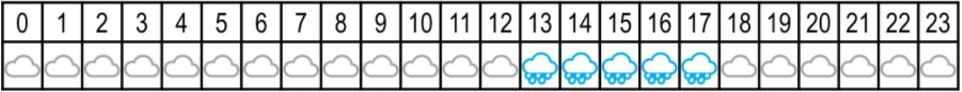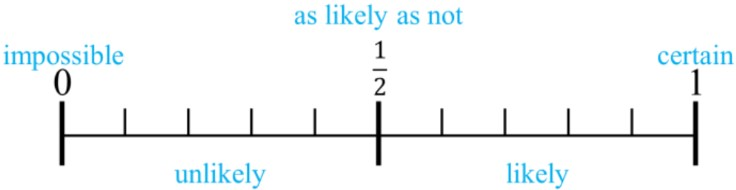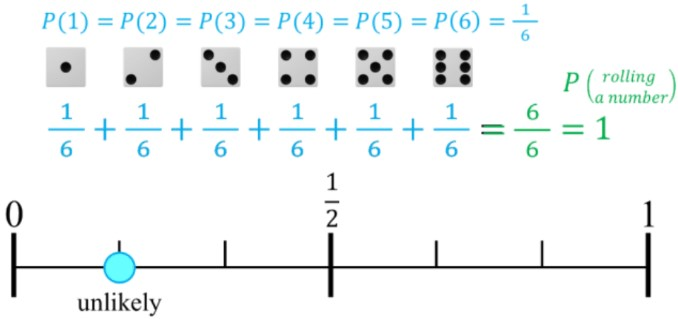# Likelihood for simple events#### Everything You Need in One Place

Homework problems? Exam preparation? Trying to grasp a concept or just brushing up the basics? Our extensive help & practice library have got you covered.#### Learn and Practice With Ease

Our proven video lessons ease you through problems quickly, and you get tonnes of friendly practice on questions that trip students up on tests and finals.#### Instant and Unlimited Help

Our personalized learning platform enables you to instantly find the exact walkthrough to your specific type of question. Activate unlimited help now!

0/3
##### Intros
###### Lessons
1. Introduction to Basic Probability for Simple Events:
2. How to describe the likelihood of something happening
3. Likelihood on a probability number line
4. Simple events on the probability line (coins, dice, spinners)
0/9
##### Examples
###### Lessons
1. Expectation and likelihood for simple events
Draw a point on the probability line for the likelihood of the event to happen. Describe using one of the words: likely, unlikely, impossible, certain, or equally likely.1. Rolling greater than 3 on a six-sided die
2. Rolling less than 7 on a six-sided die
3. Rolling the number 6 on a six-sided die
4. Rolling the number 8 on a six-sided die
5. Rolling greater than 2 on a six-sided die
2. Likelihood and probability: word problem
On a weather app, it shows the weather forecast for tomorrow with hourly weather predictions (for 24 hours). It will rain some time in the day.1. List all the possible outcomes for the weather at any time tomorrow.
2. Write the probabilities for each possible outcome. Are the outcomes equally as likely?
3. If you went outside at any random time tomorrow, how likely will you need to bring an umbrella?
4. If the weather forecast for the next day has the same outcomes as tomorrow, but they will be equally as likely to happen. How many hours out of the day will it be predicted to rain?
###### Topic Notes

In this lesson, we will learn:

• What is the likelihood of different probability events?
• The likelihood of an event can either be: impossible, unlikely, as likely as not, likely, or certain
• How to understand likelihood using a probability line (probability number line)

Notes:

• The probability of an event is a value of how likely it is to happen. We can quantify the chances of something happening using a probability fraction: $P$(event) = $\frac{number\,outcomes\,wanted}{total\,number\,possibilities}$

• The likelihood of an event can be described using the following phrases:
• Impossible - it will never happen
• Unlikely - low chances of happening (less than half the chances)
• As likely as not - just as likely to happen as to not happen (even chances; exactly half the chances)
• Likely - high chances of happening (more than half the chances)
• Certain - it will always happen (happen for sure)

• How does the value of the probability fraction represent the likelihood of an event?
• It can tell us how likely or unlikely something is to happen.
• The tool we can use to help us understand likelihood is the probability number line (probability line)• The probability line goes from 0 to 1 because the smallest probability fraction for any event is 0 out of any number of outcomes. The greatest probability fraction for any event is that it always happens (100% of the time = fraction of 1 whole).

• Ex. For rolling a six-sided die, there is a 1 in 6 chance to land on any numbered side. Rolling any of the six numbers has a low chance (all the outcomes are unlikely events).
• If you add all the probability fractions of all outcomes, the answer will be 1 whole (considering every single possible result)